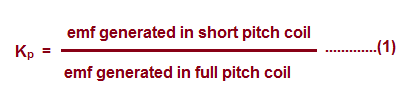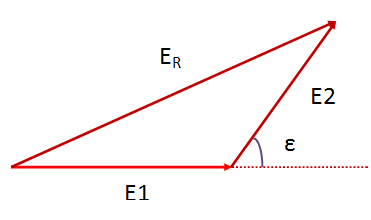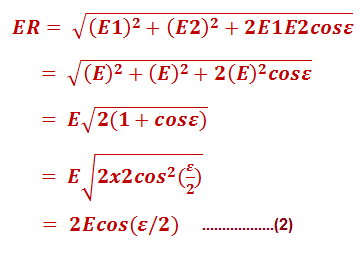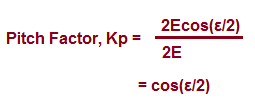# Pitch Factor or Coil Span Factor and Winding Factor

### Pitch Factor or Coil Span Factor

Pitch Factor or Coil Span Factor is definite as the ratio of emf generated in short pitch coil to the emf generated in full pitch coil. It is denoted by Kp and its value is always less than unity. This factor basically represents the effect of short pitch winding on generated emf across the winding terminals of electrical machine.

As per the definition, the formula for pitch factor is given as below.#### Calculation of Pitch Factor or Coil Span Factor

To calculate the value of pitch factor, first of all we need to calculate the value of emf generated in short pitch coil and that in full pitch coil. Let us now calculate the emf generated in a short pitch coil. Figure below shows a short pitch coil.Let E1, E2 and ER be the emf induced in coil side lying under North Pole, South Pole and resultant of emf generated in both the active lengths of coil.  As the two coil sides are separated in space by an angle of (180-ε) i.e. the coil span is (180-ε), therefore the emf induced in these coil sides will also be separated by this angle. This means, the angle between E1 and E2 phasor will be equal to ε as shown in figure below.Since the magnitude of emf generated in both the coil sides are equal, therefore E1 = E2 = E (say). As the resultant emf ER is the phasor sum of E1 and E2, thereforeAbove expression gives the emf induced in a single turn short pitch coil with chording angle ε. Since the chording angle for full pitch coil is 0 degree, therefore from the above expression (2),

emf generated in a full pitch coil = 2E

Thus from (1),Hence, pitch factor or coil span factor Kp = Cos(ε/2).

For a full pitch coil, the value of pitch factor is unity whereas for its value is also unity for concentrated coils.

The pitch factor or coil span factor nth harmonics is given as

Kp = Cos (nε/2)

### Winding Factor

Winding factor is defined as the ratio of emf generated in short pitch distributed coil to the emf generated in a concentrated coil. It is denoted by Kw.

The emf induced in a coil having N turns is given as

E = √2πKpKdfNØ ……………..(3)

where Kp and kd are pitch and distribution factor respectively.

In electrical machine, the winding are generally short pitch coils distributed along the periphery of stator slots. Therefore, to account for short pitch coil and its distribution in the stator slots, two factors Kp and kd are introduced in the expression of generated emf in (3). There are various advantages of short pitch coils. You are suggested to read “Advantage and Disadvantage of Short Pitch Winding” to understand the need for short pitch winding in electrical machine.

The emf generated in a concentrated coil having N number of turn is given as

E’ = √2πfNØ ……………..(4)

Thus from the definition of winding factor and (3) & (4),

Kw = KpKd

Thus winding factor can also be defined as the product of pitch or coil span factor and distribution factor. It value is less than 1.

Winding factor for nth harmonics is given as the product of pitch factor and distribution factor for nth harmonics. Therefore, winding factor for nth harmonics Kwn is give as below:

Kwn = KpnKdn

A short video to quickly understand the pitch factor and winding factor.

This site uses Akismet to reduce spam. Learn how your comment data is processed.